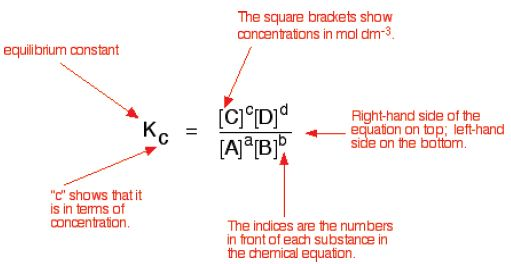# Equilibrium Constant in Electro-Chemistry

Every reversible system has its own position of equilibrium under any given set of conditions. For any given reversible reaction at equilibrium, the ratio of products to unreacted reactants remains constant. The numerical value of this ratio is called the equilibrium constant for the given reaction.

The equilibrium constant Kc

The equilibrium-constant expression for any reaction is obtained from the balanced chemical equation as follows:

aA + bB ↔ cC + dD

For the general equation above, the equilibrium constant expression would be:Also know as the Law of mass action – this expression has been determined experimentally and shown to fit all reversible reactions.

Example: For the following reaction:

N2 (g) + 3H2 (g) ↔ 2NH3 (g)

Equilibrium constant is equal to:

Kc = [NH3]2 / {[N2] x [H2]3}

The stoichiometric coefficients in the balanced equation always become the powers to which the concentrations are raised.